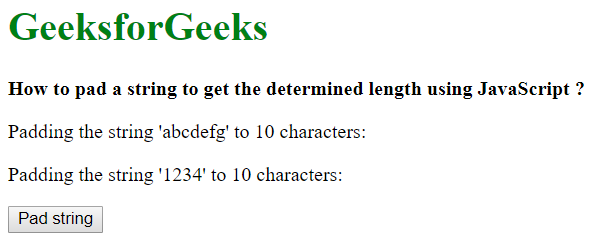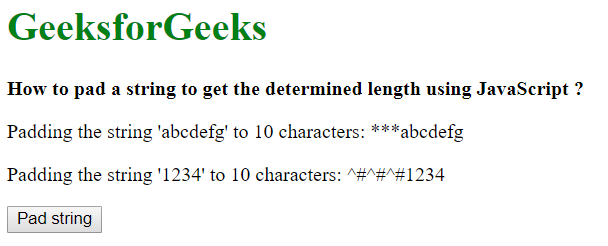# How to pad a string to get the determined length using JavaScript ?

Method 1: Using the padStart() method: The padStart() method can be used to pad a string with the specified characters to the specified length. It takes two parameters, the target length, and the string to be replaced with. The target length is the length of resulting string after the current string has been padded. The second parameter is the characters that the string would be padded.

If a number is to be padded, it has to be first converted into a string by passing it to the String constructor. Then the padStart() method is used on this string.

Syntax:

`String(strToPad).padStart(padLength, padChar)`

Example:

 ` ` `<``html``> ` ` `  `<``head``> ` `    ``<``title``> ` `        ``How to pad a string to get the determined ` `        ``length using JavaScript ? ` `    `` ` ` ` ` `  `<``body``> ` `    ``<``h1` `style``=``"color: green"``>GeeksforGeeks ` `     `  `    ``<``b``>  ` `        ``How to pad a string to get the determined ` `        ``length using JavaScript ? ` `    `` ` `     `  `    ``<``p``> ` `        ``Padding the string 'abcdefg' to 10 characters: ` `        ``<``span` `class``=``"output"``> ` `    `` ` `     `  `    ``<``p``> ` `        ``Padding the string '1234' to 10 characters: ` `        ``<``span` `class``=``"output-2"``> ` `    `` ` ` `  `    ``<``button` `onclick``=``"pad()"``>Pad string ` `     `  `    ``<``script` `type``=``"text/javascript"``> ` `     `  `        ``function pad() { ` `            ``example1 = "abcdefg"; ` `            ``example2 = 1234; ` ` `  `            ``prepended_out = String(example1).padStart(10, '*'); ` `            ``prepended_out2 = String(example2).padStart(10, '^#'); ` ` `  `            ``document.querySelector('.output').textContent ` `                    ``= prepended_out; ` `                     `  `            ``document.querySelector('.output-2').textContent ` `                    ``= prepended_out2; ` `        ``} ` `    `` ` ` ` ` `  `                     `

Output:

• Before clicking the button:• After clicking the button:Method 2: Custom function using repeat() and slice() method: The function has to be created with three arguments, the characters to be padded with, the length of the final padded string and the string to be padded.

The character to be padded with and the string to be padded is first converted into a string. This is done by using the String() constructor and passing both the values to it. These are stored in two variables, padChar and originalString. The actual number of characters to be padded is determined by subtracting the length of the string needed with the length of the characters to be padded with. This is stored in a new variable, padLeft.
The pad string that is added in front of the original string is created with the repeat() method. The repeat() method returns a new string which contains the specified number of copies of the string on which it was called. It accepts one parameter which is the number of copies to make. This method is called on the padChar string and the padLeft value is passed in this parameter. It creates the copies required for the string to be added in front of the original string. This is stored in the variable padString.
A new string is created by concatenating the padString and the originalString. The slice() method is used on this new string to make the length equal to the length required. This is done by passing a negative value of the padLength. The final string is then returned.

Example:

 ` ` `<``html``> ` ` `  `<``head``> ` `    ``<``title``> ` `        ``How to pad a string to get the determined ` `        ``length using JavaScript ? ` `    `` ` ` ` ` `  `<``body``> ` `    ``<``h1` `style``=``"color: green"``>GeeksforGeeks ` `     `  `    ``<``b``>  ` `        ``How to pad a string to get the determined ` `        ``length using JavaScript ? ` `    `` ` `     `  `    ``<``p``> ` `        ``Padding the string 'abcdefg' to 10 characters: ` `        ``<``span` `class``=``"output"``> ` `    `` ` `     `  `    ``<``p``> ` `        ``Padding the string '1234' to 10 characters: ` `        ``<``span` `class``=``"output-2"``> ` `    `` ` ` `  `    ``<``button` `onclick``=``"pad()"``>Pad string ` `     `  `    ``<``script` `type``=``"text/javascript"``> ` `     `  `        ``function padStr(padChar, padLength, originalString) { ` ` `  `            ``// Convert the pad character and original ` `            ``// string to String ` `            ``padChar = String(padChar); ` `            ``originalString = String(originalString); ` ` `  `            ``// Calculate the length of padding characters ` `            ``padLeft = padLength - originalString.length; ` ` `  `            ``// Create the pad string ` `            ``padString = padChar.repeat(padLeft); ` ` `  `            ``// Add the pad string to the original string ` `            ``// slice it to the padLength if it exceeds ` `            ``// the pad length specified ` `            ``newString = (padString +  ` `                        ``originalString).slice(-padLength); ` `            ``return newString; ` `        ``} ` ` `  `        ``function pad() { ` `            ``example1 = "abcdefg"; ` `            ``example2 = 1234; ` ` `  `            ``prepended_out = padStr('*', 10, example1); ` `            ``prepended_out2 = padStr('^#', 10, example2); ` ` `  `            ``document.querySelector('.output').textContent  ` `                    ``= prepended_out; ` `             `  `            ``document.querySelector('.output-2').textContent ` `                    ``= prepended_out2; ` `        ``} ` `    `` ` ` ` ` `  ` `

Output:

• Before clicking the button:• After clicking the button:My Personal Notes arrow_drop_upIm listening

If you like GeeksforGeeks and would like to contribute, you can also write an article using contribute.geeksforgeeks.org or mail your article to contribute@geeksforgeeks.org. See your article appearing on the GeeksforGeeks main page and help other Geeks.

Please Improve this article if you find anything incorrect by clicking on the "Improve Article" button below.